Label Encoding vs One Hot Encoding

We need numerical data in data science techniques such as machine learning and deep learning models. We start our analysis with categorical and numerical data types. When preparing the data for the model, we drop some categorical data types if we don’t need them, or we use some techniques such as regex and get numerical values. I refer to non-numeric data such as text, object, datetime, etc. with “categorical data”. There will always be some columns we need and, there won’t be any numerical values we can get in regex or another function. Therefore, to use data science techniques, we need to convert this non-numerical data to numerical data.

First of all, we must decide whether these qualitative data are nominal or ordinal. This point is important. It will define the style of our approach. If there is a hierarchy among data, it is ordinal. If there is no hierarchy among the data, it is nominal.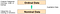Qualitative Data

Take car colors for example; there is no hierarchy, the car can be black, white, or red. It doesn’t make any order between cars. These are nominal data.

Now let’s look at the ordinal data, there are shirts in the store and the shirts have sizes such as large, medium, and small. There is a rank. Large is bigger than medium. These are ordinal data.

Encoding Methods

There are some encoding methods, but the most common and the easiest to implement are Label Encoding and One Hot Encoding.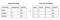Label Encoding and One Hot Encoding

1 — Label Encoding

Label encoding is mostly suitable for ordinal data. Because we give numbers to each unique value in the data. If we use label encoding in nominal data, we give the model incorrect information about our data. The model algorithm can act as if there is a hierarchy among the data.Label Encoding for Nominal Data

Let’s look at the example. There is a column with bridge types. It is nominal. Because there is no ranking among bridge types. In this example, label encoding has been implemented, and with this new column, the model algorithm can act as if there is a hierarchy between bridge types.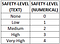Label Encoding for Ordinal Data

This is the correct use case. There is a Safety-Level column. There is a hierarchy between data, it is ordinal. Medium is low from high, so it may take 2 for medium and 3 for high. We can implement label encoding and it won’t be a problem for the model algorithm.

There are different options for applying label encoding to data. Some examples;

`from sklearn.preprocessing import OrdinalEncoderfrom sklearn.preprocessing import LabelEncoder`

OrdinalEncoder is for 2D data, LabelEncoder is for 1D data. For details visit https://datascience.stackexchange.com/questions/39317/difference-between-ordinalencoder-and-labelencoder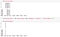For Data and Notebook https://github.com/NavarraB/DataScience/tree/master/Other

2 — One Hot Encoding

One hot encoding is the common one and the best option, especially for nominal data. We can also use it for ordinal data. It doesn’t matter if there is a hierarchy or not.

One hot encoding converts the non-numeric column to columns containing 1s and 0s.

In this method, a non-numerical column is converted into n * columns. n refers to unique values in a non-numerical column.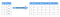One Hot Encoding

In the example above, the color column has 3 unique values; red, blue, and green. It turned into 3 columns after the one hot encoding was applied. One column for each value; color_red, color_blue, and color_green. The blue colored IDs are 2 and 4. In our converted dataframe, note the lines 2 and 4; color_red and color_green are 0 but color_blue is 1.

It can slows down models because it creates multiple columns. More columns mean less performance and more training time. It’s better to use label encoding if we have ordinal data if we don’t need it.

There are two methods to implement one hot encoding;

`from sklearn.preprocessing import OneHotEncoder`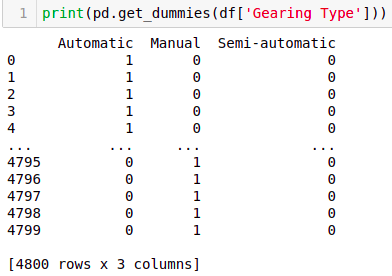One Hot Encoding with Pandas get_dummies

We can use these two methods but applying get_dummies is easier than Scikit-Learn OneHotEncoder and the get_dummies method has very useful parameters. We covered Pandas get_dummies method at this link.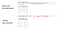Two Methods of One Hot Encoding

Conclusion

As a result, machine learning models are mathematical models that use algorithms that work with numerical data types, and neural networks also work with numerical data types. Therefore, we need to transform categorical data into meaningful numerical data.

There are some techniques we have seen above for obtaining numerical data from non-numeric data. But there are essentially two results we can get, label encoding or one hot encoding.

Check the data, decide the encoding method and apply the function.

Data Scientist | Machine Learning Proficiency | Industrial Engineer

## More from Hasan Ersan YAĞCI

Data Scientist | Machine Learning Proficiency | Industrial Engineer